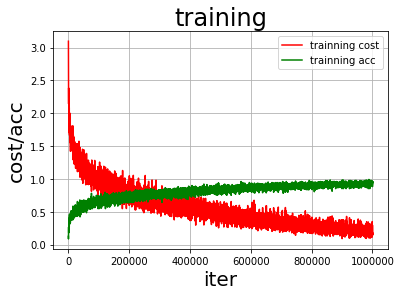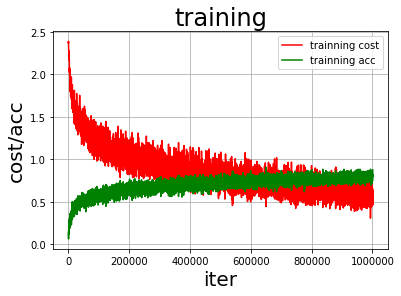## 百度机器学习训练营笔记——问题回答## 问题 1：网络结构

def convolutional_neural_network(img):
print('输入层的shape：', img.shape)
conv_pool_1 = fluid.nets.simple_img_conv_pool(
input=img,
filter_size=5,
num_filters=20,
pool_size=2,
pool_stride=2,
act="relu")
print('第一层卷积池化层输出shape：', conv_pool_1.shape)
conv_pool_1 = fluid.layers.batch_norm(conv_pool_1)
conv_pool_2 = fluid.nets.simple_img_conv_pool(
input=conv_pool_1,
filter_size=5,
num_filters=50,
pool_size=2,
pool_stride=2,
act="relu")
print('第二层卷积池化层输出shape：', conv_pool_2.shape)
conv_pool_2 = fluid.layers.batch_norm(conv_pool_2)
conv_pool_3 = fluid.nets.simple_img_conv_pool(
input=conv_pool_2,
filter_size=5,
num_filters=50,
pool_size=2,
pool_stride=2,
act="relu")
print('第三层卷积池化层输出shape：', conv_pool_3.shape)
prediction = fluid.layers.fc(input=conv_pool_3, size=10, act='softmax')
print('全连接层输出shape：', prediction.shape)
return prediction


• 输入 shape：(N,C_{in},H_{in},W_{in})
• 卷积核 shape：(C_{out},C_{in},H_f,W_f)

• 输出 shape：(N,C_{out},H_{out},W_{out})

• 输入 shape：(N,C,H_{in},W_{in})
• 池化 shape：(1,1,ksize,ksize)

H_{out}=\frac{H_{in}-ksize}{stride}+1

W_{out}=\frac{W_{in}-ksize}{stride}+1

• 输出 shape：(N,C,H_{out},W_{out})

psize=C_{out}*C_{in}*ksize*ksize

• 第一层的卷积层输出 shape：(128, 20, 28, 28)，参数大小为：20*3*5*5=1500
• 第一层的池化层输出 shape：(128, 20, 14, 14)
• 第二层的卷积层输出 shape：(128, 50, 10, 10)，参数大小为：50*20*5*5=25000
• 第二层的池化层输出 shape：(128, 50, 5, 5)
• 第三层的卷积层输出 shape：(128, 50, 1, 1)，参数大小为：50*50*5*5=62500
• 第三层的池化层输出 shape：(128, 50, 1, 1)
• 最后的全连接层输出 shape：(128, 10)，参数大小为：50*10=500
• 总参数大小：1500+25000+62500+500=89500

输入层的shape： (-1, 3, 32, 32)



• 参数的更新，使得每层的输入输出分布发生变化，称作 ICS（Internal Covariate Shift）
• 差异 hui 会随着网络深度增大而增大
• 需要更小的学习率和较好的参数进行初始化

• 可以使用较大的学习率
• 可以减少对参数初始化的依赖
• 可以拟制梯度的弥散
• 可以起到正则化的作用
• 可以加速模型收敛速度EnforceNotMet: Due to the settings of padding(0), filter_size(5), dilation(1) and stride(1), the output size is less than 0, please check again. Input_size:1